## Thursday, March 24, 2011

### Arween's Great Big Book Of Integers

Chapter 1 : Grade 7 Integer Review“When subtracting something that isn’t there use a zero pair”

Examples:-3 - (-7)=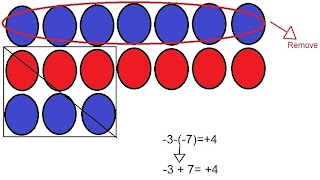-3 - 7 =3 - 7 =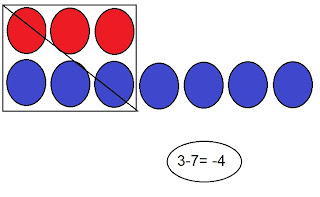3 + 7 =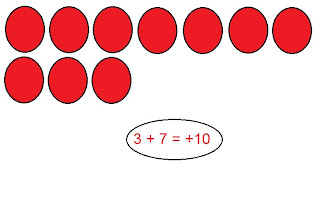-3 + 7 =Chapter 2 : Multiplying Integers
Sign rule: when you are multiplying integers and you have 0 or even numbers of negatives, the answer is positive. When you are multiplying integers and you have odd numbers of negatives, the answer is negative.

Examples:
(+2) x (+3)=(+2) x (-3)=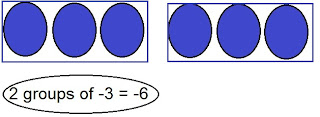(-2) x (+3)=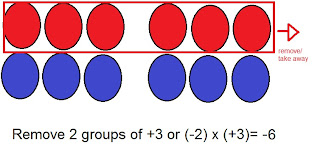(-2) x (-3)=Chapter 3 : Dividing Integers

Partitive Division is how many groups are there in a number.
6 ÷ 2 =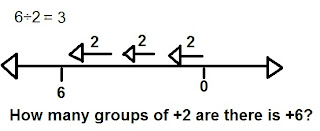Quotative division is how to share a number into groups
6 ÷ 2 =Sign rule:
Even: when numbers of negative integers are even the answer is positive
Odd: when numbers of negative integers are odd the answers is negative

6 ÷ 2 = 3 There are no negative integers so that means the quotient is positive.
-6 ÷ (-2) = 3 There are two (even) negative integers that means the quotient is positive.
(-6) ÷ 2 = -3 There is a negative integer (odd) that means the quotient is negative.
6÷(-2) = -3 There’s one negative integer that means the quotient is negative.

Chapter 4 : Order of Operations with Integers

(+5) x (-3) + (-6) ÷ (+3)
-15 + (-6) ÷ (+3)
-15 + -2
-17
When answering Order of Operations remember B.E.M.D.A.S
B = Brackets
E = Exponent
M = Multiplication
D = Division